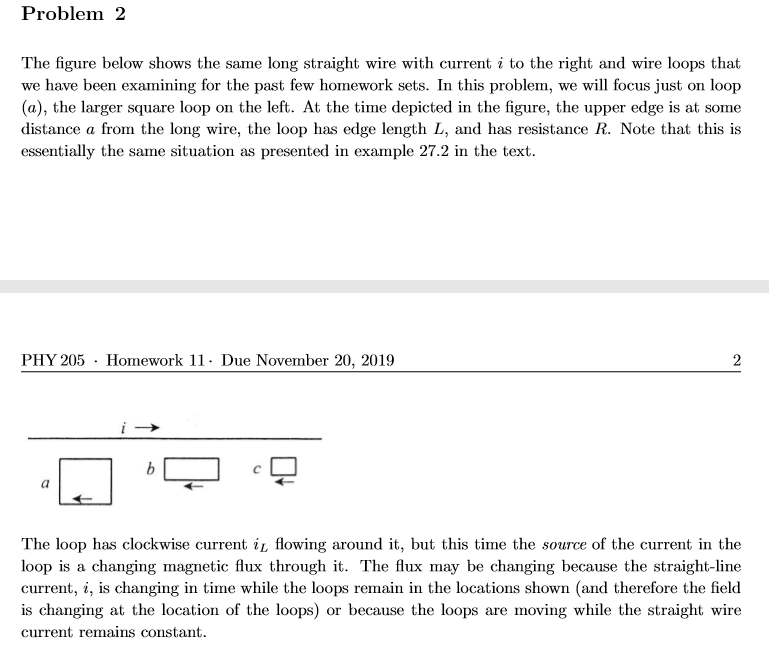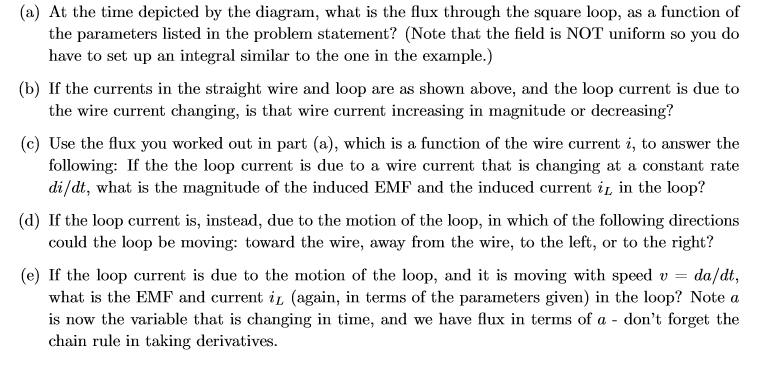# Problem 2The figure below shows the same long straight wire with current i to the right and wire loops thatwe have been examining for the past few homework sets. In this problem, we will focus just on loop(a), the larger square loop on the left. At the time depicted in the figure, the upper edge is at somedistance a from the long wire, the loop has edge length L, and has resistance R. Note that this isessentially the same situation as presented in example 27.2 in the textPHY 205Homework 11- Due November 20, 20192bThe loop has clockwise current iL flowing around it, but this time the source of the current in theloop is a changing magnetic flux through it. The flux may be changing because the straight-linecurrent, i, is changing in time while the loops remain in the locations shown (and therefore the fieldis changing at the location of the loops) or because the loops are moving while the straight wirecurrent remains constant (a) At the time depicted by the diagram, what is the flux through the square loop, as a function ofthe parameters listed in the problem statement? (Note that the field is NOT uniform so you dohave to set up an integral similar to the one in the example.)(b) If the currents in the straight wire and loop are as shown above, and the loop current is due tothe wire current changing, is that wire current increasing in magnitude or decreasing?(c) Use the flux you worked out in part (a), which is a function of the wire current i, to answer thefollowing: If the the loop current is due to a wire current that is changing at a constant ratedi/dt, what is the magnitude of the induced EMF and the induced current i in the loop?(d) If the loop current is, instead, due to the motion of the loop, in which of the following directionscould the loop be moving: toward the wire, away from the wire, to the left, or to the right?(e) If the loop current is due to the motion of the loop, and it is moving with speed v =what is the EMF and current il (again, in terms of the parameters given) in the loop? Note ais now the variable that is changing in time, and we have flux in terms of a - don't forget thechain rule in taking derivatives.da/dt,

Question
18 views

Could you answer A-C, Thank youhelp_outlineImage TranscriptioncloseProblem 2 The figure below shows the same long straight wire with current i to the right and wire loops that we have been examining for the past few homework sets. In this problem, we will focus just on loop (a), the larger square loop on the left. At the time depicted in the figure, the upper edge is at some distance a from the long wire, the loop has edge length L, and has resistance R. Note that this is essentially the same situation as presented in example 27.2 in the text PHY 205 Homework 11- Due November 20, 2019 2 b The loop has clockwise current iL flowing around it, but this time the source of the current in the loop is a changing magnetic flux through it. The flux may be changing because the straight-line current, i, is changing in time while the loops remain in the locations shown (and therefore the field is changing at the location of the loops) or because the loops are moving while the straight wire current remains constant fullscreenhelp_outlineImage Transcriptionclose(a) At the time depicted by the diagram, what is the flux through the square loop, as a function of the parameters listed in the problem statement? (Note that the field is NOT uniform so you do have to set up an integral similar to the one in the example.) (b) If the currents in the straight wire and loop are as shown above, and the loop current is due to the wire current changing, is that wire current increasing in magnitude or decreasing? (c) Use the flux you worked out in part (a), which is a function of the wire current i, to answer the following: If the the loop current is due to a wire current that is changing at a constant rate di/dt, what is the magnitude of the induced EMF and the induced current i in the loop? (d) If the loop current is, instead, due to the motion of the loop, in which of the following directions could the loop be moving: toward the wire, away from the wire, to the left, or to the right? (e) If the loop current is due to the motion of the loop, and it is moving with speed v = what is the EMF and current il (again, in terms of the parameters given) in the loop? Note a is now the variable that is changing in time, and we have flux in terms of a - don't forget the chain rule in taking derivatives. da/dt, fullscreen
check_circle

Step 1

From the Ampere’s law, the relation between the, current flowing in a wire I to the magnetic field B produced by it is given by,

Step 2

Here, μo is the permeability of free space, I is the value of the current flowing in the wire, B is the magnetic field which is produced at a distance, and dl is the line element of the loop enclosing the wire.

For the mentioned problem, the magnetic flux ΦB at an arbitrary distance x from the wire carrying time varying current I(t) within the square loop is expressed at follows.

Refer to the figure below,

Step 3

Here, the cross symbol indicates magnetic field pointing into the screen.

Consider an infinitesimal width of the length dx where the magnetic fi...

### Want to see the full answer?

See Solution

#### Want to see this answer and more?

Solutions are written by subject experts who are available 24/7. Questions are typically answered within 1 hour.*

See Solution
*Response times may vary by subject and question.
Tagged in

### Electromagnetic Induction